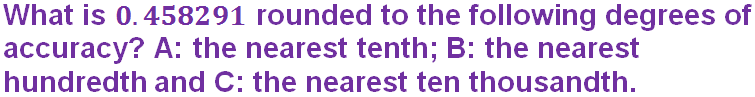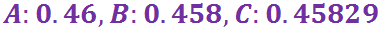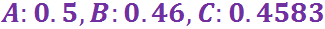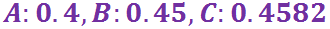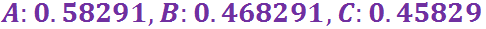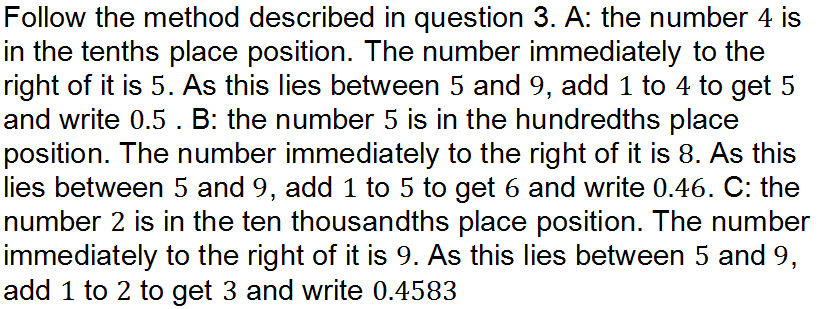Can you get ten out of ten in this quiz on decimals?

# Decimals (F)

The decimal number system allows us to write fractions of a whole number as tenths, hundredths and so on. A decimal point separates the whole number part from the fraction part (although in many countries they use a decimal comma, can you name any of these countries?) This Maths GCSE quiz will help you become more confident working with decimals.

There are a number of key skills that you should master when working with decimals. The first, and perhaps most important, is to understand the value each digit has. For example, in the number 2.467, the digit ‘4’ actually represents 4 tenths, and the ‘6’ is 6 hundredths. When reading a decimal number, each digit after the decimal point should be read individually. This will help you recognise the correct place value.

When performing addition and subtraction with decimals, line each number up in columns according to their place value. Multiplication and division of decimals is a bit trickier, so make sure you get lots of practice in.

Another key aspect is rounding a number to a stated number of decimal places. Here you have to recognise when to round up, and when to leave the final digit alone.

Don’t forget that decimals are just a different way of writing fractions that have a denominator in powers of 10. All fractions can be written as a decimal, simply divide the numerator by the denominator. Only special cases will result in a nice, terminating decimal – do you know how to recognise these?

Question 1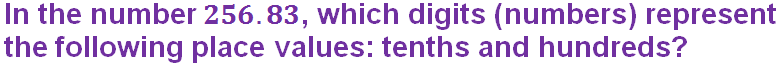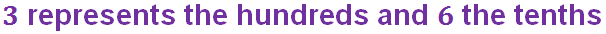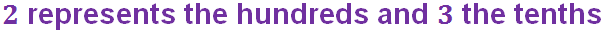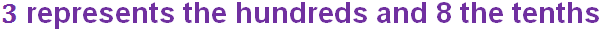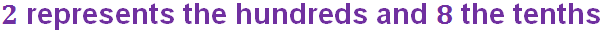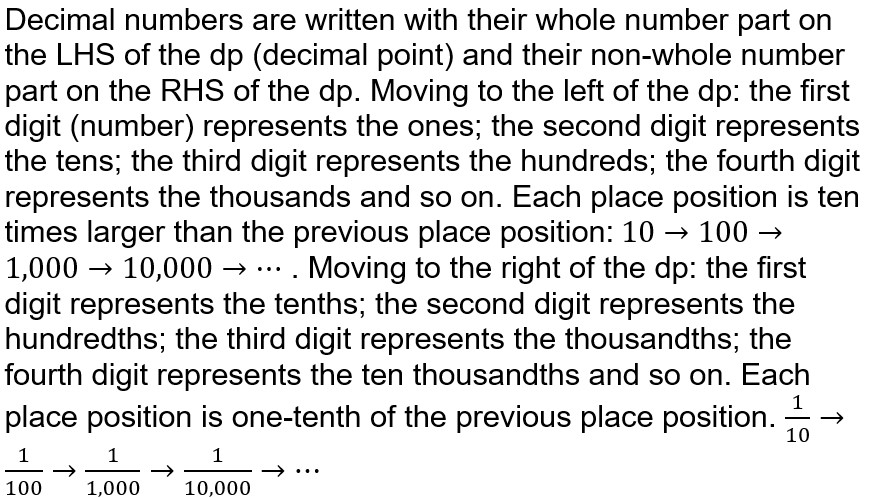Question 2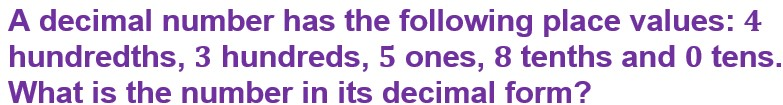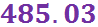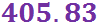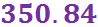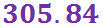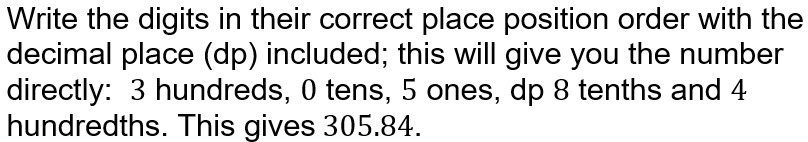Question 3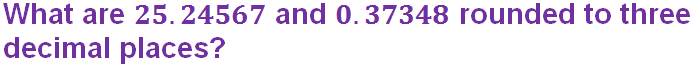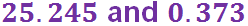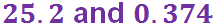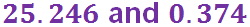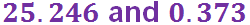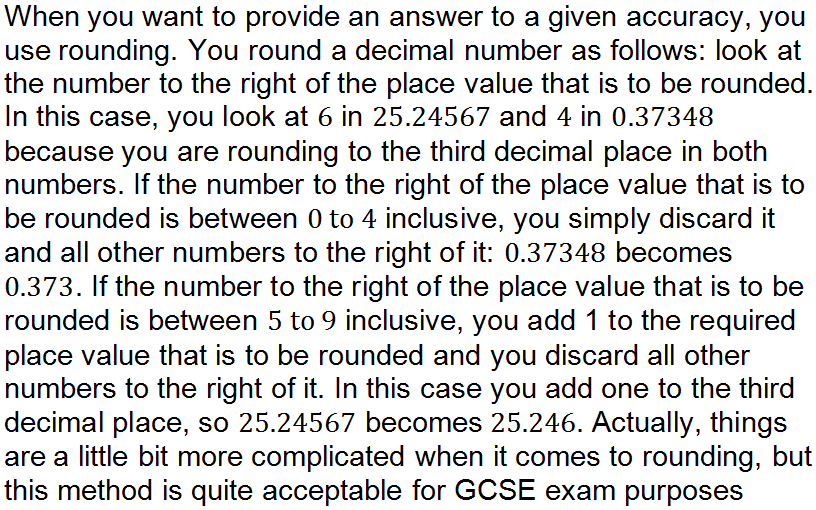Question 4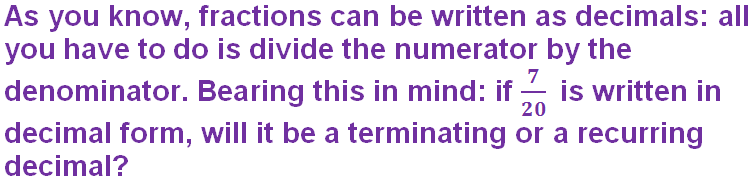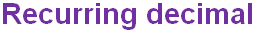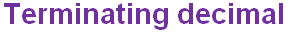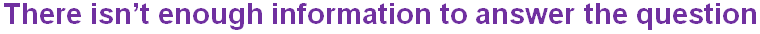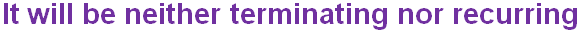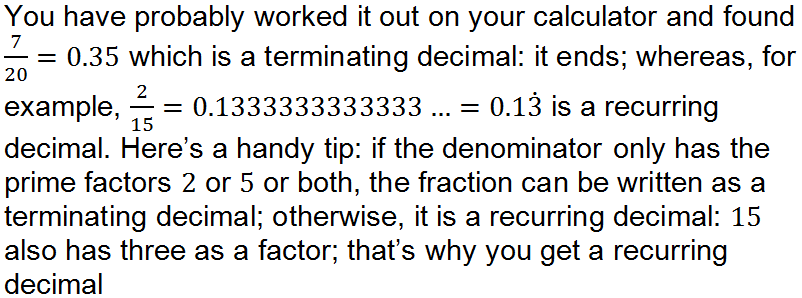Question 5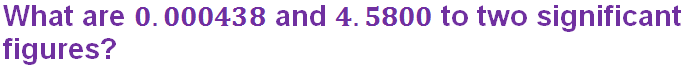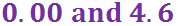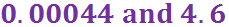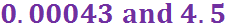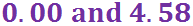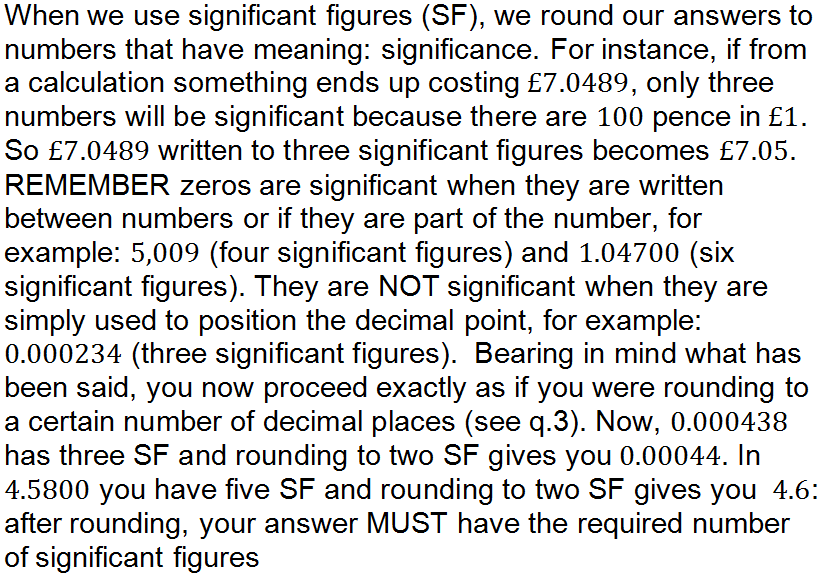Question 6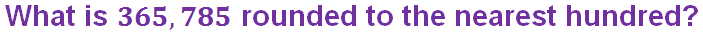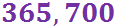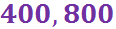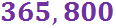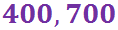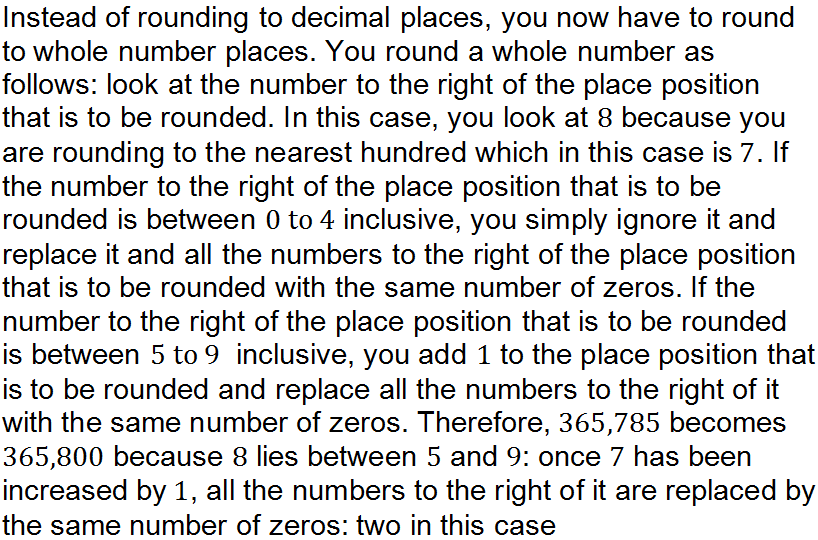Question 7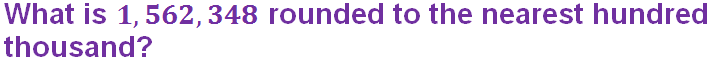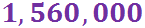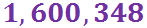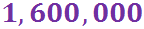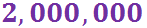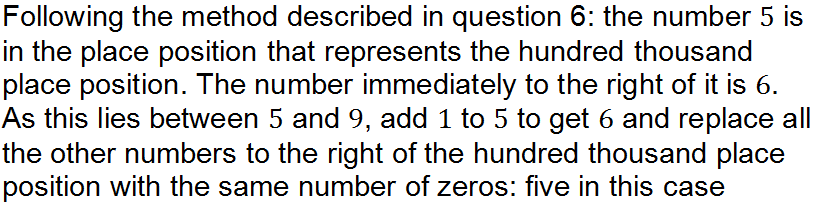Question 8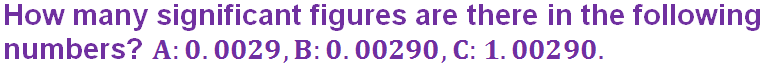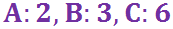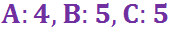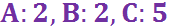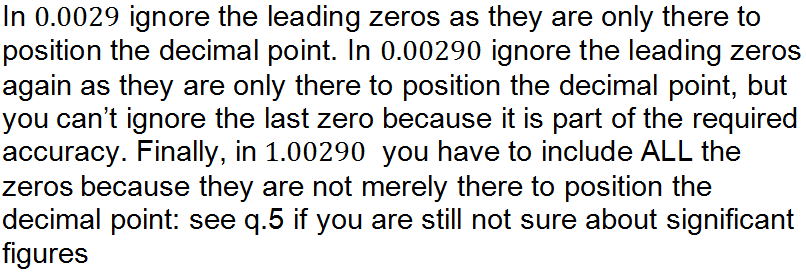Question 9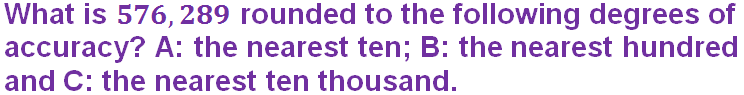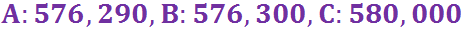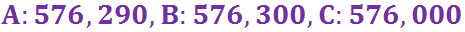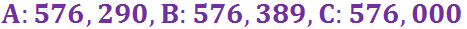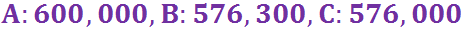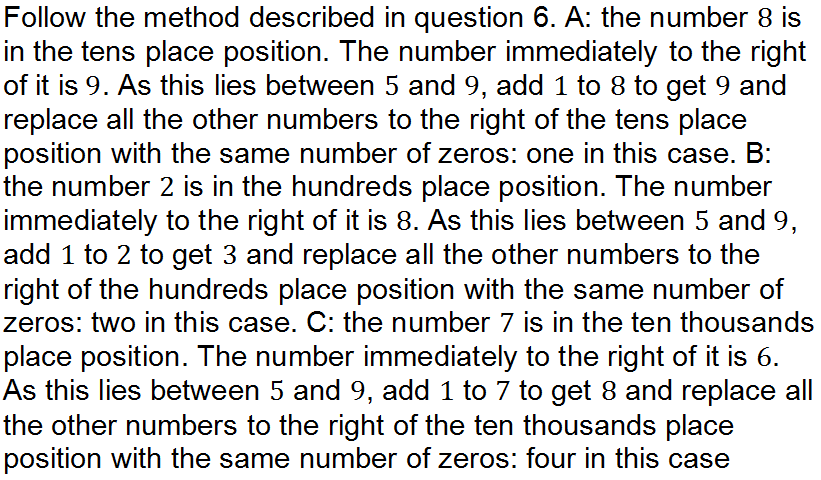Question 10